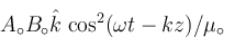Courses

# Maxwell's Equation MCQ Level - 1

## 10 Questions MCQ Test Topic wise Tests for IIT JAM Physics | Maxwell's Equation MCQ Level - 1

Description
This mock test of Maxwell's Equation MCQ Level - 1 for Physics helps you for every Physics entrance exam. This contains 10 Multiple Choice Questions for Physics Maxwell's Equation MCQ Level - 1 (mcq) to study with solutions a complete question bank. The solved questions answers in this Maxwell's Equation MCQ Level - 1 quiz give you a good mix of easy questions and tough questions. Physics students definitely take this Maxwell's Equation MCQ Level - 1 exercise for a better result in the exam. You can find other Maxwell's Equation MCQ Level - 1 extra questions, long questions & short questions for Physics on EduRev as well by searching above.
QUESTION: 1

### A capacitor (parallel plate) is being charged at a constant rate dθ/dt = b. If S is the area of the plates and d is separation between them, displacement current is

Solution:

Displacement current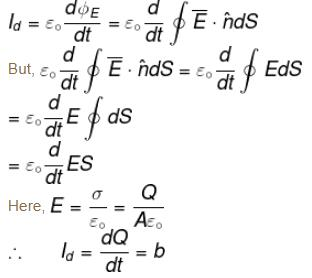QUESTION: 2

### A constant current I is flowing through a cylindrical conductor, the direction of Poynting vector is

Solution:

Let us suppose that direction of I of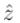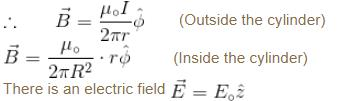Which drives the current.
∴  Direction of Poynting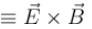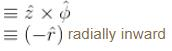The correct answer is: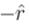QUESTION: 3

### If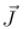is current density and ρ is the electric charge density then the equation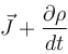represents

Solution:

If I is current,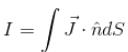then where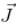is known as current density.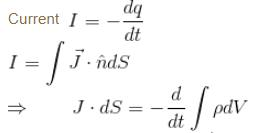By divergence theorem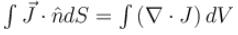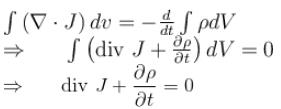This is known as equation of continuity.
The correct answer is: continuity equation

QUESTION: 4

Which one of the following Maxwell’s equations implies the absence of magnetic monopoles?

Solution: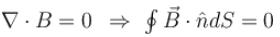Hence, it satisfies that there is no magnetic monopole.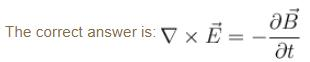QUESTION: 5

If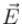is an electric field and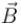is magnetic induction, then the energy per unit area per unit time in electromagnetic field is given by

Solution:

The correct answer is: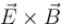QUESTION: 6

The total energy stored in electromagnetic field is

Solution:

Total energy stored in electromagnetic field is equal to sum of the energy due to the electric field (Ee) and the energy due to magnetic field (EH)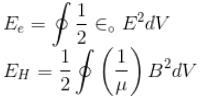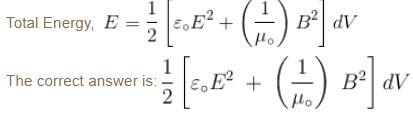QUESTION: 7

What is the Poynting vector at the surface of a long cylindrical wire of radius R, length L carrying a current I, when its ends are kept at a potential difference of V ?

Solution:

The rate of flowing energy per unit area along a plane perpendicular to the direction of the wave motion is called Poynting vector denoted by letter S and is given as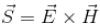Thus, Poynting vector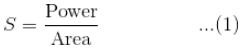Curved surface area of its cylinder = 2πrL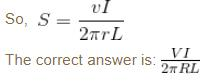QUESTION: 8

With an electromagnetic wave propagating in space, the total electromagnetic energy in a certain volume V can be written as which form ?

Solution:

The correct answer is: Essentially the rate at which at electric field is doing work on charges in the volume  i.e.,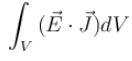QUESTION: 9

A plane electromagnetic wave is given by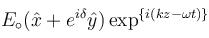At a given location, the number of times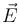vanishes in 1s is

Solution:

The plane electromagnetic wave is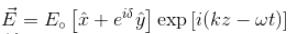The frequency of the wave is f = ω/2π
Hence,  vanishes ω/2π in 1s which is independent of δ.

The correct answer is: an integer near ω/2π and is independent of δ

QUESTION: 10

The electric and magnetic fields in the space are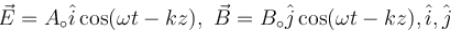being unit vectors in x and y directions respectively. The Poynting vector in this region is

Solution:

The rate of transmission of electromagnetic energy per unit area through a plane perpendicular to the direction of propagation of the electromagnetic waves is known as Poynting vector denoted by letter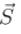and is given as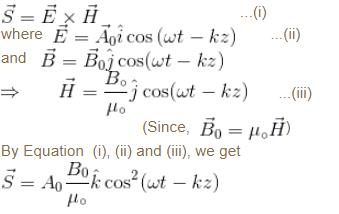The correct answer is: